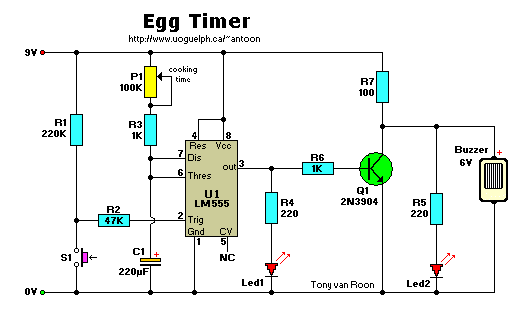```Parts List:
R1 = 220K                     P1 = 100K
R2 = 47K                      U1 = NE555/LM555
R3,R6 = 1K                Led1,Led2 = Leds, red/green
R4,R5 = 220                  Buzzer = 6V
R7 = 100                      Q1 = 2N3904
C1 = 220uF/16V

This circuit sounds a buzzer after a preset time with P1 using a common 555
timer IC.  Excellent to cook your eggs perfectly.

Have fun building or bread-boarding this circuit and don't hesitate to
experiment on it!
```